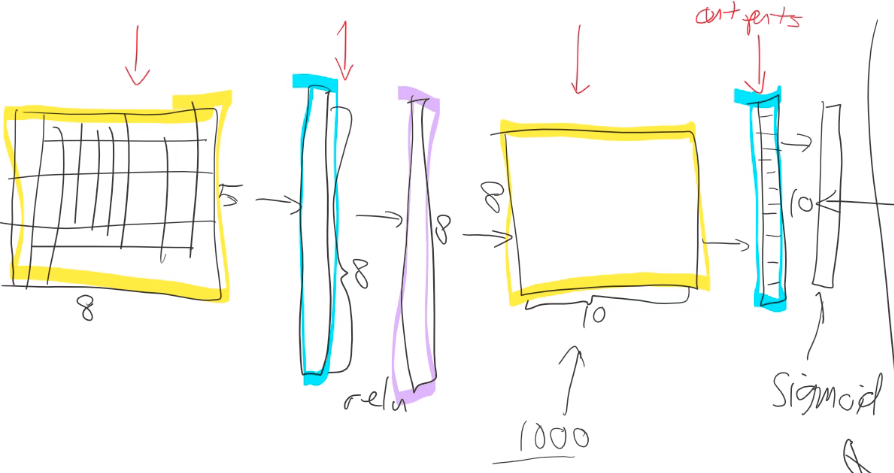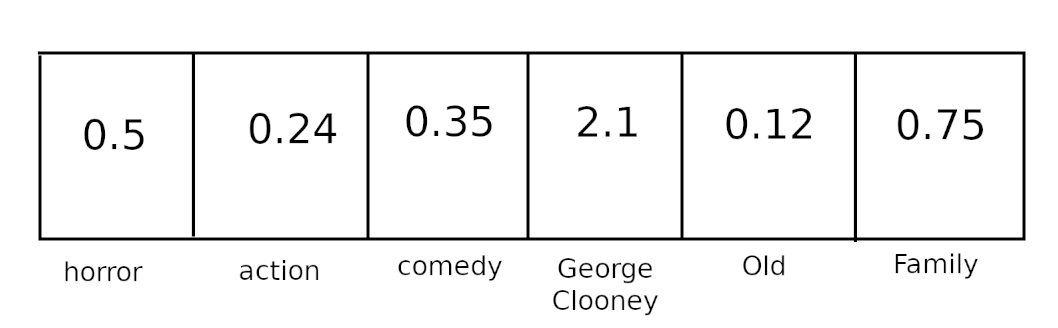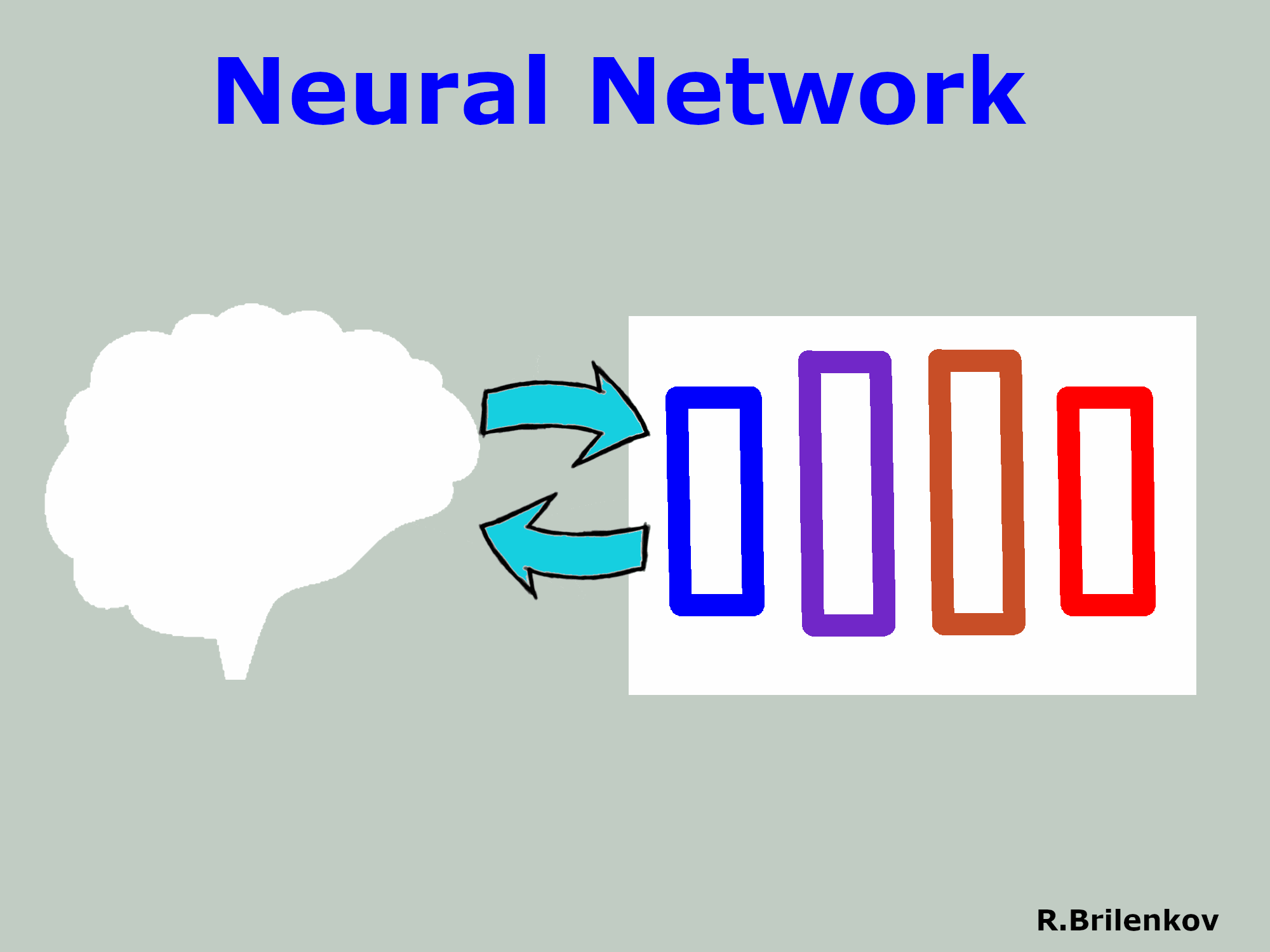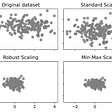# Fast.ai Lesson 5 notes — Part 1 v3

## Fine-tuningThere is also 1000 outputsThis could be a row vector for some movie. Weights tell for example how much comedy, or George Clooney there is in the movie.
`movies = pd.read_csv(path/'u.item', delimite='|', header=None,           names=['movieId','title','date','N','url',*[f'g{i}' for i          in range(19)]])`
`movies = pd.read_csv(path/'u.item',delimite='|', encoding='latin-1',          header=None, names=['movieId','title','date','N','url',*         [f'g{i}' for i in range(19)]])`
`movie_w = learn.weight(top_movies, is_item=True)movie_w.shapeOUTPUT: torch.Size([1000, 40])movie_pca = movie_w.pca(3)movie_pca.shapeOUTPUT: torch.Size([1000, 3])`

## Weight decay

`x_train,y_train,x_valid,y_valid = map(torch.tensor,(x_train,y_train,x_valid,y_valid))`
`x_train = torch.tensor(x_train)y_train = torch.tensor(y_train)x_valid = torch.tensor(x_valid)y_valid = torch.tensor(y_valid)`
`train_ds = TensorDataset(x_train,y_train)valid_ds = TensorDataset(x_valid,y_valid)`
`data = DataBunch.create(train_ds, valid_ds, bs=64)`
`x,y = next(iter(data.train_dl))x.shape,y.shapeOUTPUT:(torch.Size([64,784]), torch.Size())`
`class Mnist_Logistic(nn.Module):    def __init__(self):        # This line is important to remember.        super().__init__()        #                  Inputs,outputs        self.lin = nn.Linear(784, 10, bias=True)    def forward(self, xb): return self.lin(xb)`
`model = Mnist_Logistic().cuda()`
`[p.shape for p in model.parameters()]`
`lr=2e-2loss_func = nn.CrossEntropyLoss()def update(x,y,lr):    wd = 1e-5    y_hat = model(x)    # weight decay    w2 = 0.    for p in model.parameters(): w2 += (p**2).sum()    # add to regular loss    loss = loss_func(y_hat, y) + w2*wd    loss.backward()    with torch.no_grad():        for p in model.parameters():            p.sub_(lr * p.grad)            p.grad.zero_()     # This is just turning PyTorch tensor into normal Python number    return loss.item()`
`mse(m(x,w),y) + wd * w²`
`2wd * w`
`wd * w`
`S_t = alpha * g + (1-alpha) * S_(t-1)`
`RMSprop = old_RMSprop * 0.9 + learning_rate * gradient²weights = old_weights - (gradient * learning_rate) / RMSprop`
`adam = (momentum * learning_rate) / sqrt(RMSprop)weights = old_weights - adam`

--

--

--

## More from Lankinen

Love podcasts or audiobooks? Learn on the go with our new app.

## Creating a Simple Rule-Based Chatbot with Python## Machine Learning Optimization techniques## ML Beginner Notes: Enable GPU Settings in Google Colab## Artificial Neural Network (NN) Explained in 5 Minutes with Animations## What is a Markov Decision Process Anyways?## Train YOLOR on Custom Data?## KNOW ALL ABOUT YOUR PURCHASES OR HOW TO RECOGNIZE “TOTAL” IN CUSTOMERS CHECK## Creating a Neural Network from Scratch Using PyTorch## Machine Learning In Healthcare## Artificial intelligence and machine learning: the revolution in quality control, inspections and…## Robust Scaling — Data preprocessing## USING DEEP LEARNING FOR MUSIC GENRE CLASSIFICATION